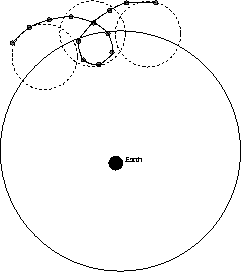Next: Units and measurements Up: Introduction Previous: Aspects of science

# Symmetry

One of the most important conceptual tools in modern science is the notion of symmetry. Although we all have an intuitive idea of what it means for something to be symmetrical (e.g. a circle has more symmetry than an irregular polygon), science requires a rigorous definition. The most commonly used definition was given by the mathematical physicist Herman Weyl.If one object has more symmetries than another, we say it is more symmetrical. For example, a square is left unchanged by any rotation through 90o, or 180o, or 270o. Such rotations are among the symmetries of the square. An irregular polygon, on the other hand will look different after being rotated through any angle. It therefore has no symmetries: the square is more symmetrical. The most symmetrical of all such geometrical shapes that we can draw on a plane is the circle. It can be rotated through any angle between 0o and 360o. Thus the circle has an (uncountable) infinity of symmetry operations that leave it unchanged. Early astronomers thought the circle to be the most perfect of geometrical shapes, and reasoned that astronomical objects must move in circles in order for the Universe to be as perfect as possible. In the Ptolemaic system, the planets were thought to revolve around the Earth, and to their dismay, astronomers found that the motions of the planets were much too complicated to be considered simple circles. In order to retain their guiding principles (the Earth as the center of the Universe, and the notion of maximum symmetry), they were forced to explain the relatively complicated apparent motion of the planets by using epicycles, or circular orbits within circular orbits.This was one of the first applications of symmetry to a problem in science. Unfortunately, it turned out to be misguided. The Ptolemaic system, which was regarded as correct for over 1400 years was wrong because its underlying hypothesis was incorrect. Using a combination of observation and mathematics, Copernicus realized that it was possible to construct a simple and elegant model for the of motion of the planets in terms of circles by assuming that they orbited the Sun and not the Earth. In the Copernican system, the planetary orbits are indeed nearly circular. This is a good illustration of how the scientific method occasionally requires a radical rethinking of the popular worldview. It was a difficult adjustment for people of Copernicus' day to accept that the Earth was not the center of the Universe.

In the above we talked about symmetries of objects, or things, such as polygons, and planetary orbits. It is possible, and in fact necessary, to apply the notion of symmetry directly to the laws of science themselves. As stated earlier, the purpose of science in general, and physics in particular is to find laws that explain nature. These laws would be impossible to discover if they weren't in some sense uniform and unchanging. For example, if the laws of science changed every day, we would never be able to figure them out. It would be like trying to determine the rules of a card game that someone was making up as they went along. So we have to assume that physical laws do not change with time. This is our first example of a symmetry of the laws of nature. It is called time translation symmetry. Similarly we must assume that the laws of physics are the same here as on the other side of the solar system, or the other side of the planet. i.e. the laws of physics must be the same everywhere. This is called, simply, translational symmetry. Finally, we assume that the laws of physics don't change if we turn around. i.e. they are independent of the direction in which we are facing. This is called rotational symmetry.

The three symmetries (translation, time translation and rotation) have long been accepted as valid symmetries of nature. Albert Einstein used a different symmetry as a motivating principle when he revolutionized our concept of the Universe with his special theory of relativity. Einstein firmly believed that the laws of physics should be the same for all observers, independent of their state of motion. For example, any experiment should have precisely the same outcome whether it is performed here on the Earth, or in a spaceship moving at constant velocity relative to the Earth. In order to retain this symmetry principle, Einstein was forced to give up one of the fundamental tenets of the Newtonian world view, namely the existence of an absolute notion of time on which all observers could agree. The special theory of relativity, which will be discussed in more detail in a later chapter, is the ultimate example of a symmetry principle leading to a radical revision of fundamental concepts. However, it should be emphasized that Einstein's special theory of relativity, and its bizarre picture of space and time, could only be fully accepted once it was confirmed by experimental observation.

Today, symmetry is a fundamental cornerstone of modern physics. By assuming that physical systems have, at least approximately, a high degree of symmetry, it is possible to radically simplify the equations that describe them. In addition, the quest for a unified description in elementary particle physics (the physics of the particles that make up the nucleus), is guided by the notion that the correct theory will be the most symmetrical theory possible. In this sense we have gone back to the Ptolemaic view.Next: Units and measurements Up: Introduction Previous: Aspects of science
modtech@theory.uwinnipeg.ca
1999-09-29